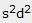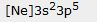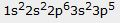# Trends on the Periodic Table MC

Which of the following elements is a metal?
Li
Si
S

Which of the following elements has the smallest ionization energy?
As
P
N

Which of the following elements has the largest atomic mass?
Sc
Ca
K

Which of the following elements is a member of the halogen family?
Cl
Ar
S

Which of the following elements has the greatest electron affinity?
P
Al
Si

Which of the following elements has the largest atomic radius?
Ga
Al
Si

Which of the following elements has the largest atomic number?
Ta
V
Nb

Which of the following elements is a member of noble gases?
Xe
I
Te

Which of the following elements has 4 energy levels?
Ge
Si
Sn

Which of the following elements is a member of alkali metals?
Li
Be
B

Which of the following elements has 6 valence electrons?
Se
As
Br

Which of the following elements is a nonmetal?
H
Na
Li

Which of the following elements is a member of transition metals?
Hg
Tl
Pb

Which of the following elements has electron distribution ending in?
Al
Mg
Na

Which of the following elements is a metalloid?
Po
Pb
Bi

Which of the following elements is gas at room temperature?
N
C
B

Which of the following elements has electron distribution ending in?
Ti
Sc
Ca

Use your periodic table to determine which of the following elements has the largest atomic number.
Rb
Na
K
Li

Use your periodic table to determine which of the following elements has the largest atomic radius.
C
N
O
F

Use your periodic table to determine which of the following elements has the largest ionization energy.
F
I
Br
Cl

Use your periodic table to determine which of the following elements has the largest electron affinity.
Cl
P
Si
S

Which of the following is the correct “noble gas” distribution for chlorine?source